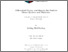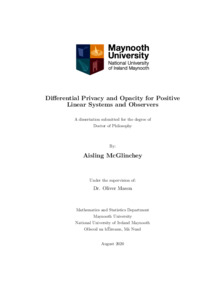# Diﬀerential Privacy and Opacity for Positive Linear Systems and Observers

McGlinchey, Aisling (2020) Diﬀerential Privacy and Opacity for Positive Linear Systems and Observers. PhD thesis, National University of Ireland Maynooth.Previewmore...Add this article to your Mendeley library

## Abstract

Positive systems are important for application such as transportation, population dynamics and epidemiology. These systems can sometimes make use of sensitive personal information, which leads to the challenge of publishing this information in a way that guarantees individuals’ privacy, while keeping the data usable. In this thesis, we focus on the problem of applying privacy constraints to positive linear systems and observers via diﬀerential privacy and opacity. After reviewing relevant notation and background, weﬁrsttackletheproblem of building a diﬀerentially private mechanism that preserves positivity. We consider two methods of constructing these mechanisms: post-processing and restriction. We present explicit formulae for the bias and the mean square error of the mechanisms constructed. We derive two results showing that bias is unavoidable for both approaches. Forpost-processing with a ramp function, we determine the optimal such function that minimises the worst case bias. We also prove the existence of an optimal post-processing function for mean square error. Throughout this thesis, a major focus is on the positive linear observer problem. Speciﬁcally, we derive bounds for both the l1 and l2 sensitivity of Luenberger observers. We also show how these bounds can be used to quantify the noise required to achieve diﬀerential privacy via the Laplace or Gaussian mechanism. We then study the optimisation problem of minimising these bounds for positive linear observers and provide methods for computing an optimal solution for diﬀerent classes of systems. In particular, we derive theoretical results describing optimal observers, in the l1 sensitivity sense, for compartmental systems with a single output and a subclass of multi-output compartmental systems. We also consider the trade-oﬀ between the l1 sensitivity bound of a positive linear observer and the rate at which it converges to the true system state. Finally, we present preliminary results on initial state opacity for positive linear systems. In particular, we consider the problem of initial state opacity when the secret and non-secret states are deﬁned by convex cones in Rn +. Farkas’ Lemma is used to derive a result characterising opacity in this case.

Item Type: Thesis (PhD) Diﬀerential Privacy; Opacity; Positive Linear Systems; Observers; Faculty of Science and Engineering > Mathematics and Statistics 13616 IR eTheses 17 Nov 2020 15:41Item control page